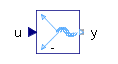Pacejka

Pacejka tire model used to determine the forces and moments developed at the tire contact patchThe Pacejka 2002 tire model, unlike the analytical Fiala and Calspan models, is based on fitting experimental data to the Pacejka's Magic Formula. Complex expressions for all tire forces and moments are computed, taking into account a wide range of physical phenomena. This model requires that numerous parameters are employed.

Note: The $\mathrm{hubFrame}$ port of the Pacejka component must be connected directly to the $\mathrm{hubFrame}$ port of another tire body component, as shown in the diagram below.Connections

 Name Description Color $\mathrm{hubFrame}$ Tire reference frame, which must be connected to a tire body component. Gray

Parameters

Note: All units are assumed to be in SI unless otherwise noted.

 Symbol Default Units Description ID ${\mathrm{Fz}}_{0}$ $5900$ $N$ Nominal normal force Fz0 ${R}_{0}$ $0.355$ $m$ Unloaded radius R0 ${V}_{0}$ $22.2$ $\frac{m}{s}$ Nominal (measured) forward speed V0 ${\mathrm{LFz}}_{0}$ $1$ - Scale factor of nominal load LFz0 ${\mathrm{LC}}_{\mathrm{xy}}$ $\left[1,1\right]$ - Scale factor of Fx,Fy shape factor LCxLCy ${\mathrm{Lμ}}_{\mathrm{xy}}$ $\left[1,1\right]$ - Scale factor of Fx, Fy peak friction coefficient LmuxLmuy ${\mathrm{LE}}_{\mathrm{xy}}$ $\left[1,1\right]$ - Scale factor of Fx, Fy curvature factor LExLEy ${\mathrm{LK}}_{\mathrm{xy}}$ $\left[1,1\right]$ - Scale factor of Fx, Fy slip stiffness LKxLKy ${\mathrm{LH}}_{\mathrm{xy}}$ $\left[1,1\right]$ - Scale factor of Fx, Fy horizontal shift LHxLHy ${\mathrm{LV}}_{\mathrm{xy}}$ $\left[1,1\right]$ - Scale factor of Fx, Fy vertical shift LVxLVy ${\mathrm{Lγ}}_{\mathrm{xyz}}$ $\left[1,1,1\right]$ - Scale factor of inclination for Fx, Fy, Mz Lgammaxyz ${L}_{\mathrm{trail}}$ $1$ - Scale factor of peak pneumatic trail Ltrail ${L}_{\mathrm{res}}$ $1$ - Scale factor for offset of residual torque Lres ${\mathrm{LX}}_{\mathrm{α}}$ $1$ - Scale factor of slip-angle influence on Fx LXalpha ${\mathrm{LY}}_{\mathrm{κ}}$ $1$ - Scale factor of longslip influence on Fy LYkappa ${\mathrm{LVy}}_{\mathrm{κ}}$ $1$ - Scale factor of longslip influence on Fy vertical shift LVykappa $\mathrm{LS}$ $1$ - Scale factor of moment arm of Fx about vertical axis LS ${\mathrm{LM}}_{\mathrm{xy}}$ $\left[1,1\right]$ - Scale factor of overturning couple and rolling resistance torque LMxLMy $\mathrm{LVMx}$ $1$ - Scale factor of Mx vertical shift LVMx ${\mathrm{PCx}}_{1}$ $1.65$ - Shape factor for longitudinal force PCx1 ${\mathrm{PDx}}_{\mathrm{1...3}}$ $\left[1,0,0\right]$ - Longitudinal friction at Fz0, force variation with load, inclination PDx1PDx3 ${\mathrm{PEx}}_{\mathrm{1...4}}$ $\left[0,0,0,0\right]$ - Longitudinal curvature at Fz0, variation with load, variation with load squared, factor while driving (causes asymmetry) PEx1PEx4 ${\mathrm{PKx}}_{\mathrm{1...4}}$ $\left[20,0,0\right]$ - Longitudinal slip stiffness at Fz0, variation with load, exponent in slip stiffness with load PKx1PKx3 ${\mathrm{PHx}}_{\mathrm{1...2}}$ $\left[0,0\right]$ - Horizontal shift of longitudinal slip at Fz0, variation with load PHx1PHx2 ${\mathrm{PVx}}_{\mathrm{1...2}}$ $\left[0,0\right]$ - Vertical shift at Fz0, variation with load PVx1PVx2 ${\mathrm{RBx}}_{\mathrm{1...2}}$ $\left[10,6\right]$ - Slope factor for combined slip Fx reduction, variation with load RBx1RBx2 ${\mathrm{RCx}}_{1}$ $1$ - Shape factor for combined slip Fx reduction RCx1 ${\mathrm{REx}}_{\mathrm{1...2}}$ $\left[0,0\right]$ - Curvature factor for combined slip Fx reduction, variation with load REx1REx2 ${\mathrm{RHx}}_{1}$ $0$ - Shift factor for combined slip Fx reduction RHx1 ${\mathrm{QSx}}_{\mathrm{1...3}}$ $\left[0,0,0\right]$ - Overturning moment coefficients QSx1QSx3 ${\mathrm{PCy}}_{1}$ $1.1058$ - Shape factor for pure lateral force PCy1 ${\mathrm{PDy}}_{\mathrm{1...3}}$ $\left[1.04910,-0.215300,-0.338480\right]$ - Lateral peak friction, variation with load, inclination squared PDy1PDy3 ${\mathrm{PEy}}_{\mathrm{1...4}}$ $\left[-0.871480,-1.5278,-0.0259870,-0.907290\right]$ - Lateral force curvature factor at Fz0, variation with load, sign of slip angle, inclination PEy1PEy4 ${\mathrm{PKy}}_{\mathrm{1...3}}$ $\left[-23.648,2.1393,-0.907290\right]$ - Maximum value of cornering stiffness, load (Fz/Fz0) at which cornering stiffness reaches its max value, variation of with inclination PKy1PKy3 ${\mathrm{PHy}}_{\mathrm{1...3}}$ $\left[-0.000178020,-0.0000349800,0.0290550\right]$ - Horizontal shift of lateral force at Fz0, variation with load, inclination PHy1PHy3 ${\mathrm{PVy}}_{\mathrm{1...4}}$ $\left[-0.000453100,0.000540830,-0.206230,-0.155680\right]$ - Vertical shift at Fz0, variation with load, inclination, inclination and load PVy1PVy4 ${\mathrm{RBy}}_{\mathrm{1...3}}$ $\left[16,0,0\right]$ - Slope factor for combined slip Fy reduction, variation with slip angle, shift term for alpha in slope factor RBy1RBy3 ${\mathrm{RCy}}_{1}$ $1$ - Shape factor for combined slip Fy reduction RCy1 ${\mathrm{REy}}_{\mathrm{1...2}}$ $\left[0,0\right]$ - Curvature factor for combined slip Fy reduction, variation with load REy1REy2 ${\mathrm{RHy}}_{\mathrm{1...2}}$ $\left[0,0\right]$ - Shift factor for combined slip Fy reduction, variation with load RHy1RHy2 ${\mathrm{RVy}}_{\mathrm{1...6}}$ $\left[0,0,0,0,1.9,0\right]$ - Longitudinal slip induced side forces at Fz0, variation with load, inclination, slip angle, longitudinal slip, and arctan of longitudinal slip RVy1RVy6 ${\mathrm{QSy}}_{\mathrm{1...4}}$ $\left[0.01,0,0,0\right]$ - Rolling resistance coefficients QSy1QSy4 ${\mathrm{QBz}}_{\mathrm{1...5}}$ $\left[14.973,-3.5918,-0.72704,-0.00777650,-0.0081978\right]$ - Pneumatic trail slope factor at Fz0, variation with load, load squared, inclination, absolute inclination QBz1QBz5 ${\mathrm{QBz}}_{\mathrm{9...10}}$ $\left[17.781,0\right]$ - Slope factors for residual torque QBz9QBz10 ${\mathrm{QCz}}_{1}$ $1.132$ - Shape factor for pneumatic trail QCz1 ${\mathrm{QDz}}_{\mathrm{1...4}}$ $\left[0.112720,-0.00267450,-0.00663320,-6.23800\right]$ - Peak pneumatic trail, variation with load, inclination, load and inclination QDz1QDz4 ${\mathrm{QDz}}_{\mathrm{6...9}}$ $\left[0.00031508,0.0002961,-0.081607,-0.08016\right]$ - Peak residual torque, variation with load, inclination, load and inclination QDz6QDz9 ${\mathrm{QEz}}_{\mathrm{1...5}}$ $\left[-1.7447,-0.58532,0,0.00292020,-2.2229\right]$ - Pneumatic trail curvature at Fz0, variation with load, load squared, sign of slip angle, sign of slip angle and inclination QEz1QEz5 ${\mathrm{QHz}}_{\mathrm{1...4}}$ $\left[0.000252220,0.0000684680,-0.0192730,0.00927610\right]$ - Pneumatic trail horizontal shift at Fz0, variation with load, inclination, load and inclination QHz1QHz4 ${\mathrm{SSz}}_{\mathrm{1...4}}$ $\left[0,0,0,0\right]$ - Nominal value of S/R0, variation with Fy, inclination, load and inclination SSz1SSz4

References

 1 H.B. Pacejka, Tire and Vehicle Dynamics, SAE International, 2002.